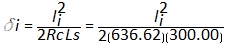### 9. Power Series Method

#### a. Equations

A more accurate way to compute spiral points is using infinite power series, Equations E-24 and E-25.Equation E-24Equation E-25

δi in Equations E-24 and E-25 is in radians; it is computed with Equation E-18.

Although both equations have an infinite number of terms, each term is substantially smaller so only the first three need be used.

Radial chords are computed using Equation E-23 and deflection angles using Equation E-26.Equation E-26

#### b. Example

Using the spiral of the previous example, set up and solve the spiral curve table.Chord Num li δi, radians xi, ft yi, ft ci, ft ai 1 60.00 0.009425 60.00 0.19 60.00 0°10'48" 2 120.00 0.037699 119.98 1.51 119.98 0°43'12" 3 180.00 0.084823 198.87 5.09 179.87 1°37'12" 4 240.00 0.150796 239.46 12.04 239.46 2°52'46" 5 300.00 0.235619 298.34 23.47 298.34 4°29'52"

Compare this curve table to the first table in Section 7e. The results of this "more exact" calculations are basically the same as those based on approximations.

Should a much longer spiral or one with a great radii difference be used, the approximations may be might introduce measurable error in which case the power series equations should be used.